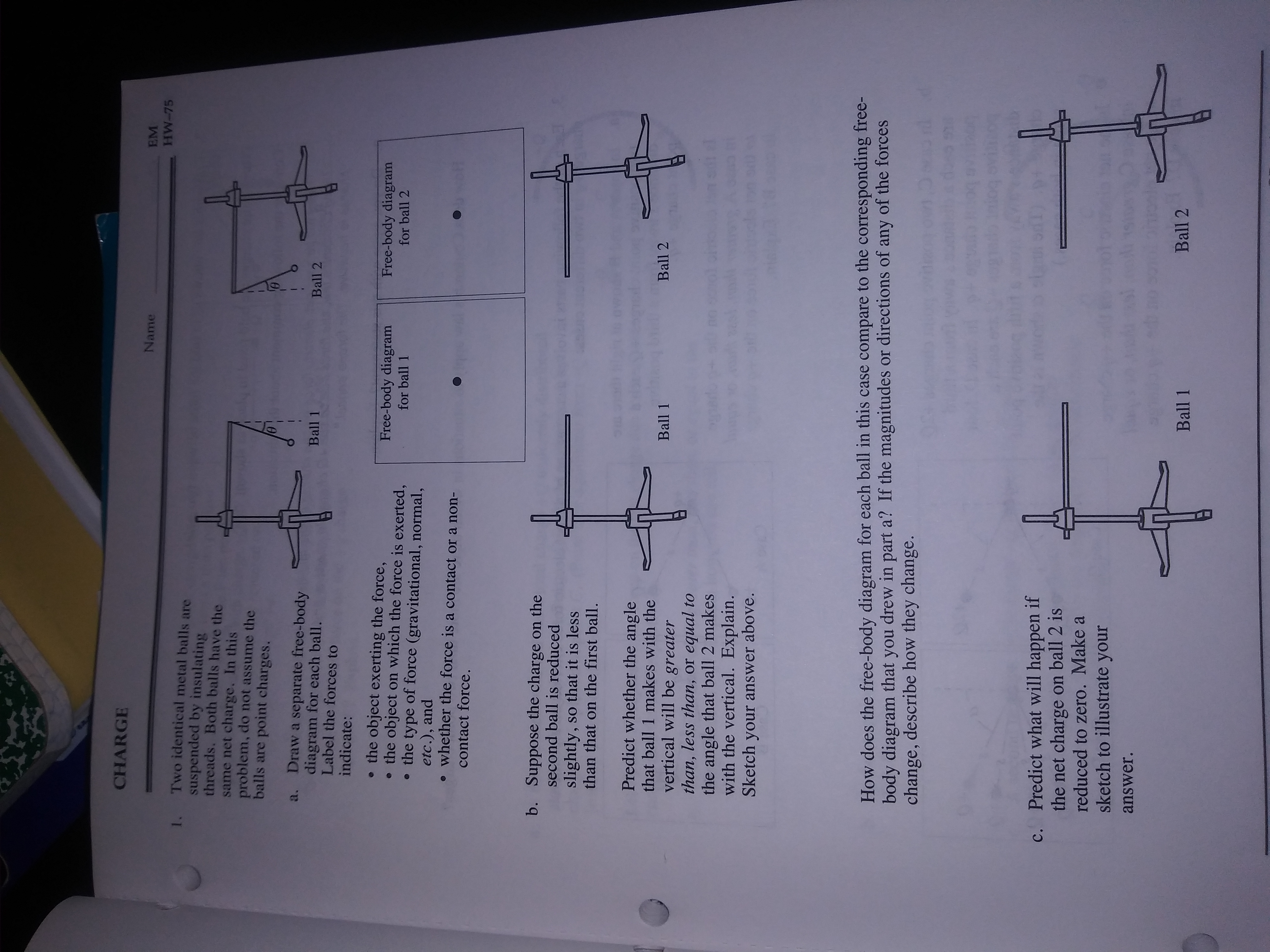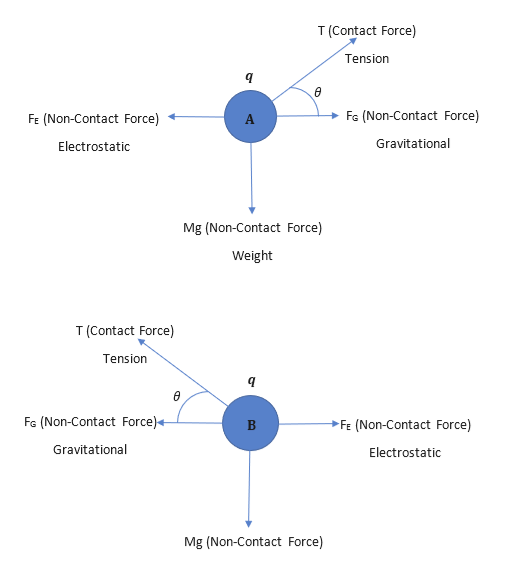# CHARGENameEMHW-75Two identical metal balls aresuspended by insulatingthreads. Both balls have the1.same net charge. In thisproblem, do not assume theballs are point charges.a. Draw a separate free-bodydiagram for each ball.Label the forces toBall 1Ball 2indicate:the object exerting the force,the object on which the force is exerted,the type of force (gravitational, normal,etc.), andwhether the force is a contact or a non-Free-body diagramFree-body diagramfor ball 1for ball 2contact force.Suppose the charge on thesecond ball is reducedb.slightly,than that on the first ball.so that it is lessPredict whether the anglethat ball 1 makes with thevertical will be greaterthan, less than, or equal tothe angle that ball 2 makeswith the vertical. Explain.Sketch your answer above.Ball 2Ball 1How does the free-body diagram for each ball in this case compare to the corresponding free-body diagram that you drew in part a? If the magnitudes or directions of any of the forceschange, describe how they change.Predict what will happen ifthe net charge on ball 2 isreduced to zero. Make ac.sketch to illustrate youranswer.Ball 2Ball 1

Question
335 views

Problem 1

a through c.help_outlineImage TranscriptioncloseCHARGE Name EM HW-75 Two identical metal balls are suspended by insulating threads. Both balls have the 1. same net charge. In this problem, do not assume the balls are point charges. a. Draw a separate free-body diagram for each ball. Label the forces to Ball 1 Ball 2 indicate: the object exerting the force, the object on which the force is exerted, the type of force (gravitational, normal, etc.), and whether the force is a contact or a non- Free-body diagram Free-body diagram for ball 1 for ball 2 contact force. Suppose the charge on the second ball is reduced b. slightly, than that on the first ball. so that it is less Predict whether the angle that ball 1 makes with the vertical will be greater than, less than, or equal to the angle that ball 2 makes with the vertical. Explain. Sketch your answer above. Ball 2 Ball 1 How does the free-body diagram for each ball in this case compare to the corresponding free- body diagram that you drew in part a? If the magnitudes or directions of any of the forces change, describe how they change. Predict what will happen if the net charge on ball 2 is reduced to zero. Make a c. sketch to illustrate your answer. Ball 2 Ball 1 fullscreen
check_circle

star
star
star
star
star
1 Rating
Step 1

Given:

Two identical metal balls with the same net charge hanging with the help of a thread separately.

Step 2

Let the ball 1 is ball A and have mass 'm' and charge 'q'. ball 2 is ball B, have the same mass 'm' and same charge 'q'. As shown in the given figure both the balls make angle of θ with the vertical.

The forces involved are Gravitational, electrostatic, tension from the thread and weight of the balls.

(a) Free body diagrams of both the balls are as follows:help_outlineImage TranscriptioncloseT (Contact Force) Tension Fa (Non-Contact Force) FE (Non-Contact Force) Gravitational Electrostatic Mg (Non-Contact Force) Weight T (Contact Force) Tension FG (Non-Contact Force) FE (Non-Contact Force) Gravitational Electrostatic Mg (Non-Contact Force) fullscreen
Step 3

(b) Let the charge on the second ball (Ball B) becomes q' such that q'<q.

Both the charges exerts equal electrostatic forces on each other irrespective of the value of the charge on them. But due to reduction in charge on ball B, the value of electrostatic force between the ball will reduce. Hence, the angle with the vertical will also ...

### Want to see the full answer?

See Solution

#### Want to see this answer and more?

Solutions are written by subject experts who are available 24/7. Questions are typically answered within 1 hour.*

See Solution
*Response times may vary by subject and question.
Tagged in

### Electric Charges and Fields